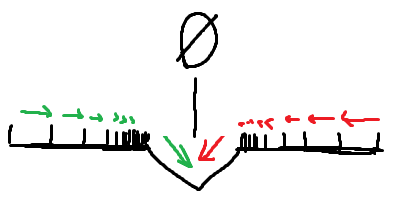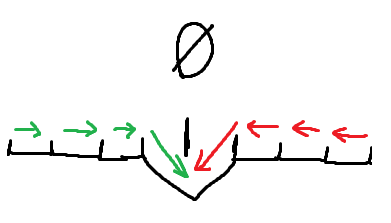# Easing Efficiency

This is a more open ended on efficiency.

``````    -- This is in a while true do
wait()
if seat.Throttle == 0 then	-- Ease to standstill
if math.abs(inputSpeed) > seat.Torque then
if inputSpeed > seat.Torque then
inputSpeed = inputSpeed - seat.Torque
else
inputSpeed = inputSpeed + seat.Torque
end
else
inputSpeed = 0
end
else	-- Increase speed
inputSpeed = math.clamp(inputSpeed + seat.Torque * seat.Throttle, seat.MaxSpeed * -0.5, seat.MaxSpeed)
end
base.BodyVelocity.velocity = base.CFrame.lookVector*inputSpeed
``````

That’s some simple code for speeding/ slowing something down.

I’m happy with setting speed, but when the throttle is idle, I want a more efficient/ less “if statement” way of easing to 0.

There’s always the option of just doing:

``````inputSpeed = inputSpeed * 0.98
``````

That would ease the number closer to 0 every cycle. The problem is that it’s inconsistent from MANUALLY trying to slow down from top speed/ speed up from reverse.What I want is there to be very little difference between manually slowing down and speeding up.
Like as follows:Have you guys found efficient ways to do this?

I believe if you add a drag value it should do what you’re looking for.

Don’t exactly quote me on the details I remember seeing this on a post but you essentially assign a ‘drag’ value and minus it from your power

1 Like

How would I make the drag go up from negative numbers, down from positive, but stop around 0?

Here’s a method that uses less if statements but isn’t really more efficient. I don’t think you’ll be able to achieve both.

``````if math.abs(inputSpeed) > seat.Torque then
inputSpeed = inputSpeed - math.sign(inputSpeed) * seat.Torque
else
inputSpeed = 0
end
``````

Here’s a single line solution as well.
`inputSpeed = inputSpeed * (1-math.min(math.abs(seat.Torque/inputSpeed), 1))`

2 Likes

I remember seeing drag from a post and I finally found it

Essentially from one of the replies I got `Force = Throttle * Power - Drag * Speed`
however I took it out of context because that makes a force ‘constant’ meaning it won’t go over a certain amount.

I was thinking for a while and thought that you could possibly get when the user stops input and grab the current speed of the vehicle with `base.Velocity.Magnitude` and see the difference between the current speed and 0 so lets say the difference is 25, that is how much you would increase the backwards force by (this is all just theoretical I have not actually tested it)